Compound Interest refers to earning or paying interest on interest. Although it can apply to both savings and loans, it is easiest to understand when thinking about savings. After each compound period, the interest earned over that period is added to the principal so that the next calculation of interest includes the original principal plus the previously earned interest.

With Simple Interest (the opposite of compound interest), interest is only calculated from the principal, and interest is not added to the principal.

This page includes a basic online compound interest calculator that you can use for simple future value problems. You can download the spreadsheet to see how compounding works from month to month.

## Compound Interest Calculator

Compound interest is used for both savings and loans, but this calculator is based on its use in calculating the future value of savings.

### Compound Interest Calculator

 Principal or Start Amount (P) Annual Interest Rate (r) % Compound Frequency (n) Annually (1) Semi-Annually (2) Quarterly (4) Bi-Monthly (6) Monthly (12) Semi-Monthly (24) Bi-Weekly (26) Weekly (52) Daily (365) Years of Growth (t) Payment (A) Payment Frequency (p) Annually (1) Semi-Annually (2) Quarterly (4) Bi-Monthly (6) Monthly (12) Semi-Monthly (24) Bi-Weekly (26) Weekly (52) Future Value (F) Total Payments Total Interest * Results might only be estimates. See caution below.

This calculator uses the following formulas and definitions:

```F = P*(1+rate)^nper + A*( ((1+rate)^nper - 1)/rate )
rate = ((1+r/n)^(n/p))-1
nper = p * t
Total Payments = A*nper
Total Interest = F - P - Total Payments
```
• r = nominal annual interest rate (decimal)
• n = number of compounding periods per year
• p = number of payment periods per year
• rate = rate per payment period
• nper = total number of payment periods
• A = an amount added to the principal at the end of each payment period

When the Payment (A) is zero, a little algebra will show that the above formula simplifies down to the basic compound interest formula:

```F = P*(1+r/n)^(n*t)
```

* Caution This calculator lets you choose Payment and Compounding combinations that don't necessarily make sense. For example, a compound frequency of Monthly and a payment frequency of Weekly don't match up (there isn't an exact number of weeks in a month). The math still gives a result, but it probably would not match what is going on from week to week in an actual savings account.

## Compound Interest Calculator for Excel

⤓ Excel (.xlsx)
For: Excel 2010 or later & Excel for iPad/iPhone

License: Personal Use (not for distribution or resale)

"No installation, no macros - just a simple spreadsheet" - by

### Description

This spreadsheet was designed as an educational tool - to help show how compound interest works for both savings and loans. The table is based on the payment frequency and shows the amount of interest added each period. The graph compares the total (cumulative) principal and payments to the balance over time.

One of the worksheets in this file is nearly identical to the online calculator above, and was used to help verify the calculations.

BETA (7/29/2019) - This spreadsheet is currently a BETA version because I haven't tested every possible input combination. Plus, people tend to use spreadsheets in ways I haven't thought of.

Update 10/30/2022 - Fixed the Daily compounding option to reference "Daily (365)"

## Compound Interest Formula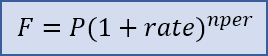The basic compound interest formula for calculating a future value is F = P*(1+rate)^nper where

• F = the future accumulated value
• P = the principal (starting) amount
• rate = the interest rate per compounding period
• nper = the total number of compounding periods

### Formula for Compounding Yearly, Monthly, Weekly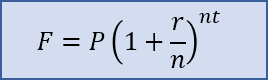The formula is often written as F = P*(1+r/n)^(n*t) with the following variables definitions:

• P = the principal amount (the initial savings or the starting loan amount)
• r = the nominal annual interest rate in decimal form. (e.g. 5% means r=0.05)
• n = the number of compound periods per year (e.g. for monthly, n=12)
• t = the time in years

This is the same as the basic formula where rate = r/n and nper = n*t. Although the math can handle a decimal value for nper, it should usually be a whole number. For example, with monthly compounding for a total of 18 months, n=12 and t=1.5 resulting in nper=12*1.5=18.

### Formula for Daily Compounding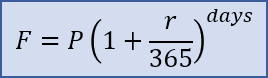For daily compounding, the value for n (number of compound periods per year) is typically 365 and you use total number of days in place of n*t like this: F = P*(1+r/365)^days. For day count conventions other than n=365, see the wikipedia article.

## How to Calculate Compound Interest in Excel

In Excel and Google Sheets, you can use the FV function to calculate a future value using the compound interest formula. The following three examples show how the FV function is related to the basic compound interest formula.

```F = P*(1+rate)^nper
F = -FV(rate,nper,,P)
F = FV(rate,nper,,-P)
```

Example 1: What is the future value of an initial investment of \$5,000 that earns 5% compounded annually for 10 years? Answer: F = 5000*(1+0.05)^10 = 8144.47. The Excel formula would be F = -FV(0.05,10,,5000) or F = FV(5%,10,,-5000). In Excel, when you add a percent sign after a number, the number is divided by 100.

Example 2: What is the future value of an initial investment of \$5,000 that earns 5% compounded monthly for 10 years? Answer: F = 5000*(1+0.05/12)^(12*10) = 8235.05. The Excel formula would be F = -FV(0.05/12,12*10,,5000) or F = FV(5%/12,12*10,,-5000).

Note For savings calculations, the FV function in Excel can be a bit confusing because if you enter the present value as a positive number, you need to negate the final result. That is because with annuity functions like FV and PV, Excel assumes that cash you pay out, such as your initial savings and deposits to savings, is represented as negative numbers.

The syntax for the FV function in Excel is FV(rate,nper,pmt,[pv],[type]). Excel solves for FV using the following equation (for rate≠0):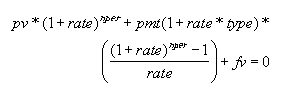Fig 1: Formula relating pv, rate, nper, pmt, fv in Excel.

When pmt=0, fv=-pv*(1+rate)^nper, so the variable P used in the standard compound interest formula relates to the Excel formula as P=-pv. Likewise, the variable A (defined below as positive for deposits to savings) relates to pmt as A=-pmt.

## Compound Interest Formula for a Series of Payments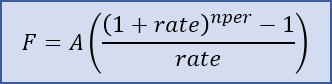For both loans and savings, we typically want to include a series of payments or deposits in our calculation, such as depositing 100 each month for 3 years. The formula for the future value of a uniform series of deposits or payments is F=A(((1+rate)^nper-1)/rate) where

• A = the payment amount, added to the principal at the end of each period
• rate = the rate per payment period
• nper = the number of payments

When the payment period matches the compound period, rate=r/n and nper=n*t.

This formula can be derived from the compound interest formula, based on the fact that the total future value is the sum of each individual payment compounded over the time remaining. If you are interested in the derivation, see Reference  at the bottom of this page.

In Excel and Google Sheets, we can use the FV function again. The formulas below show how the FV function relates to the standard formula. Note that for now we aren't including a principal amount.

```F = A*(((1+rate)^nper-1)/rate)
F = -FV(rate,nper,A)
F = FV(rate,nper,-A)
```

Example 3: If I deposit \$1000 at the end of each year, and my investment earns 4% annually, what is the future value at the end of 5 years? Answer: =1000*(((1+0.04)^5-1)/0.04) = 5416.32. The Excel formula would be F = -FV(0.04,5,1000) or F = FV(4%,5,-1000).

Note These formulas assume that the deposits (payments) are made at the end of each compound period. According to Figure 1, this means that type=0 (the default for the FV function). If I wanted to deposit \$1000 at the beginning of each year for 5 years, the FV function in Excel allows me to calculate the result as =FV(4%,5,-1000,,1) where type=1. Just remember that the type argument has to do with the timing of the deposits (A), not the principal (P).

### Future Value for both Principal and a Series of Payments

When you start with a non-zero principal amount and you make a series of payments, the resulting formula is just the sum of the two formulas described above. The FV function lets you include both the payment amount and the principal as follows:

```F = P*(1+rate)^nper + A*(((1+rate)^nper-1)/rate)
F = -FV(rate,nper,A,P)
F = FV(rate,nper,-A,-P)
```

Example 4: If my starting savings is \$4000 and I earn a whopping 6% compounded annually and I deposit \$200 at the end of each year, what is the future value at the end of 5 years? Answer: =4000*(1+0.06)^5 + 200*(((1+0.06)^5-1)/0.06) = 6480.32. The Excel formula would be F = -FV(0.06,5,200,4000).

The table below shows how the calculations work each compound period. The table starts with an initial principal of P0=4000. The next rows shows that at the end of the first year, the interest is calculated a i1=rate*P0. The new principal is P1=P0+i1+A. This process continues until the end of year 5, where P5=6480.32 (the same value we calculated with the compound interest formula).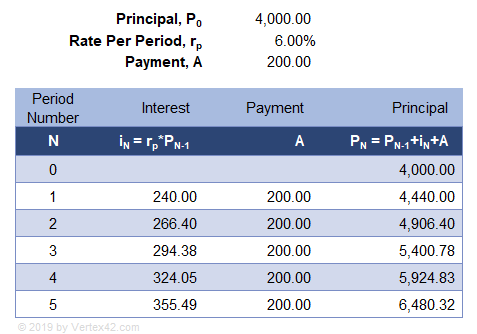## Formula for Rate per Payment Period (when Compound Period ≠ Payment Period)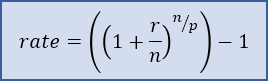The calculator at the top of the page allows you to choose a compound frequency that is different from the payment frequency. The Rate Per Payment Period is calculated using the formula rate = ((1+r/n)^(n/p))-1 and the total number of periods is nper = p*t where

• r = the nominal annual interest rate in decimal form
• n = the number of compound periods per year
• p = the number of payment periods per year
• t = the time in years

These values for rate and nper can then be used in the compound interest formulas mentioned above.

A common example where this formula is needed is for a savings account where the interest is compounded daily but deposits are only made monthly. To approximate what the bank is doing, you can use n=365 (Compound Frequency = Daily) and p=12 (Payment Frequency = Monthly).

Another real-world example is the Canadian mortgage where the compounding is semi-annual (2 times per year) and the payments are monthly (12 per year).

## Compound Interest Formula for Loans

Traditional amortized loans use the same formulas as those defined above for savings, except that the loan amount is represented as a negative value for the starting principal, P. Payment amounts (A) are still positive values.

### How to Calculate the Loan Payment

For fully amortized loans, you typically need to calculate the payment amount (A) that will make the Future Value zero (F=0) after a specific number of years. The formula for the payment amount is found by solving for A using the formula from Figure 1. In Excel, you can use the PMT function. Note that if the loan is for \$3000, P=-3000).

```AF=0 = (-P*(1+rate)^nper) / (((1+rate)^nper - 1)/rate)
AF=0 = PMT(rate,nper,P))
```

Example 5: If I apply for a loan for \$3000 with a rate of 6% compounded annually, what is my payment if I want to pay it off completely in 5 years? Answer: A = (-(-3000)*(1+0.06)^5) / (((1+0.06)^5-1)/0.06) = 712.1892. The Excel formula is A = PMT(0.06,5,-3000).

The table below uses the exact same equations as the savings example, except that the principal is P0=-3000 and the payment, A, was calculated so that the future value is zero after year.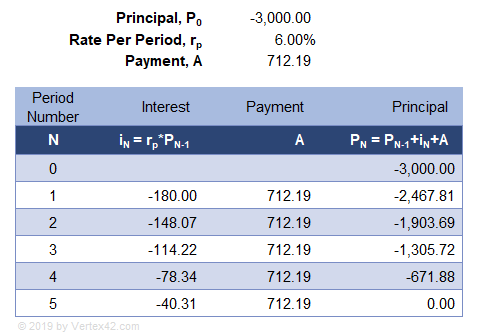### Am I paying "Interest on Interest" if my loan payments are on time?

That is an interesting debate. Here are the two competing arguments.

Argument #1: Yes. The table in Example 5 clearly shows that the new principal is calculated by adding the interest and the payment to the previous principal. The formulas are exactly the same as the savings example, except that you are starting with a negative principal. The formulas show that interest IS added to the principal, which satisfies the definition of compound interest, and that explains why you can use the compound interest formulas in traditional loan calculations.

Argument #2: No. For an amortized loan, the payment isn't actually 100% principal. Instead, you must first pay the amount of interest that you are charged, and the rest of your payment is applied to the principal. (This is how almost all amortized loans are worded). You are paying the interest first, so no interest is added to the principal. Therefore, you are not paying interest on interest if your payments are enough to completely pay the interest charged each period.

I was in the camp of Argument #2 for many years, and it wasn't until creating these compound interest calculators that I realized Argument #2 is just legal jargon - a way to claim you aren't paying interest on interest. Saying that the payment is only partially principal because the interest is first subtracted from the payment means that the formula for the new principal, PN, would just be written with parentheses as PN=PN-1+(iN+A). The parentheses tell us to first add the interest (a negative value in this case) to the loan payment. Then, the result is added to the principal. Does that actually change the final value? Of course not.

Argument #2 then says "Yeah, but your payment is enough to completely pay the amount of interest charged, so no interest is actually added to the principal. Therefore, you aren't paying interest on interest."

Argument #1 replies with "Yeah, but without affecting the result, the math allows me to consider my payment to be applied to the original principal, with the interest added afterward. Thus, I AM paying interest on interest."

Argument #2 would then say "Our definition of the loan payment means that you are forced to add the amounts in parentheses first, so we are allowed to say we aren't adding interest to the principal."

Which argument is correct? Or, could they both be correct? Ultimately, the fact is that the compound interest formulas calculate the same result for Example 5, regardless.

What does this mean for the borrower?

Ultimately, the take away is that part of your regular loan payment is being used to pay off interest. To take advantage of the compound interest formula, the borrower should make additional principal-only payments. Learn how debt payoff is similar to an investment.

Note Even a so-called Simple Interest Loan requires the payments to be applied first to the accrued interest before they can be applied to the principal. So, even though the daily interest accrual is based on simple interest, the amortization is still based on the compound interest formula.

### References

Disclaimer: This information on this page is for educational purposes only. We do not guarantee the results or the applicability to your unique financial situation. You should seek the advice of qualified professionals regarding financial decisions.